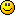#### Topic: Widner’s Support/Resistance Oscillators WSO / WRO

Through my way to find a different types of indicators types i find that indicator WSO/WRO
Its a different type of osc build using fractals not price it gives fractals a new use "not to be a breakout indicator only" but also used as a trend indicator
i find it will be useful if we have such indicator in our arsenal

attached here an article shows how that indicator working  it sound promisingregards

#### Re: Widner’s Support/Resistance Oscillators WSO / WRO

here is another link shows also how indicator could be used  along with MT4 indicator

http://mechanicalforex.com/2011/02/the-indicator-series-dr-widners-support-and-resistance-oscillators.html

#### Re: Widner’s Support/Resistance Oscillators WSO / WRO

How to insert an image in the body of post?????

#### Re: Widner’s Support/Resistance Oscillators WSO / WRO

http://www.flickr.com/photos/85383260@N … /lightbox/

levels are
0 ,100, 66.6667 , 83.3333 , 50 , 16,6667 , 33.3333
and these all levels we can see so we cannot see level 40 and so on
its like a stears not actual numbers like other osc
So they could replaced by 1,2,3,4,5 and 6 levels

sup go up
sup go down
sup unchanged
sup at level 100
sup at level 0
sup at level 66.6
sup at level 83.3
sup at level 50
sup at level 16.6
sup at level 33.3
res go up
.
.
.etc

#### Re: Widner’s Support/Resistance Oscillators WSO / WRO

hello

Any luck to code that indicator!!!!

NB: attached is the WSO WRO oscillator with moving average

#### Re: Widner’s Support/Resistance Oscillators WSO / WRO

Hello ahmedalhoseny,
Thanks for sharing great indicator! This is the first time I hear about it. Sounds interesting.

#### Re: Widner’s Support/Resistance Oscillators WSO / WRO

Hello Kazik its a nice indicator and i wish one of coders here convert it to FSB code soon

wish it helps

#### Re: Widner’s Support/Resistance Oscillators WSO / WRO

Hi ahmedalhoseny,
Do you know when this indicator will be converted to FSB?

#### Re: Widner’s Support/Resistance Oscillators WSO / WRO

Hello footon
could you manage to code this wso wro indicator !!

#### Re: Widner’s Support/Resistance Oscillators WSO / WRO

Yes it would be mostly welcome#### Re: Widner’s Support/Resistance Oscillators WSO / WRO

It's a pity no one devotes energy to create such indicator. It could make a huge difference. Support and resistance are one of the best tools out there...
I would do it myself but I need to learn how to code in C (I guess).

#### Re: Widner’s Support/Resistance Oscillators WSO / WRO

Hello
Its an old topic and i wish we can finish this tricky indicator

attached my trial for the indicator but it gives a different results it seams need some tweaking inside the code
Any help from pop or footon  will be appreciated

#### Re: Widner’s Support/Resistance Oscillators WSO / WRO

Which source you used? Honestly I don't see any similarities between those 2 sources above and your trial... It's very hard to give pointers and advice.

#### Re: Widner’s Support/Resistance Oscillators WSO / WRO

sources in ur mail

#### Re: Widner’s Support/Resistance Oscillators WSO / WRO

Here is source in AFL (amibroker thing) for interested parties:

``````/////////////////////////
// RSI of WRO and WSO ///
////////////////////////

TimeFrameSet(60*in1Minute);

// WRO - WIDNER'S RESISTANCE OSCILLATOR
p1=-4;
p2=9;
r1=ValueWhen(Ref(L,p1)==LLV(L,p2),Ref(L,p1),1);
r2=ValueWhen(Ref(L,p1)==LLV(L,p2),Ref(L,p1),2);
r3=ValueWhen(Ref(L,p1)==LLV(L,p2),Ref(L,p1),3);
r4=ValueWhen(Ref(L,p1)==LLV(L,p2),Ref(L,p1),4);
r5=ValueWhen(Ref(L,p1)==LLV(L,p2),Ref(L,p1),5);
r6=ValueWhen(Ref(L,p1)==LLV(L,p2),Ref(L,p1),6);
r1m=Max(0,Min(1,int(r1/C)));
r2m=Max(0,Min(1,int(r2/C)));
r3m=Max(0,Min(1,int(r3/C)));
r4m=Max(0,Min(1,int(r4/C)));
r5m=Max(0,Min(1,int(r5/C)));
r6m=Max(0,Min(1,int(r6/C)));
WRO=100*(1-(r1m+r2m+r3m+r4m+r5m+r6m)/6);
Plot(WRO,"",colorGreen,styleDashed);

// WSO - WIDNER'S SUPPORT OSCILLATOR
p1=-4;
p2=9;
s1=ValueWhen(Ref(H,p1)==HHV(H,p2),Ref(H,p1),1);
s2=ValueWhen(Ref(H,p1)==HHV(H,p2),Ref(H,p1),2);
s3=ValueWhen(Ref(H,p1)==HHV(H,p2),Ref(H,p1),3);
s4=ValueWhen(Ref(H,p1)==HHV(H,p2),Ref(H,p1),4);
s5=ValueWhen(Ref(H,p1)==HHV(H,p2),Ref(H,p1),5);
s6=ValueWhen(Ref(H,p1)==HHV(H,p2),Ref(H,p1),6);
s1m=Max(0,Min(1,int(s1/C)));
s2m=Max(0,Min(1,int(s2/C)));
s3m=Max(0,Min(1,int(s3/C)));
s4m=Max(0,Min(1,int(s4/C)));
s5m=Max(0,Min(1,int(s5/C)));
s6m=Max(0,Min(1,int(s6/C)));
WSO=100*(1-(s1m+s2m+s3m+s4m+s5m+s6m)/6);
Plot(WSO,"",colorRed,styleDashed);

// AVERAGES + RSI
x1=EMA(WRO,5);
x2=EMA(WRO,10);
x3=EMA(WRO,15);
x4=(x1+x2+x3)/3;
x5=RSIa(x4,5);

y1=EMA(WSO,5);
y2=EMA(WSO,10);
y3=EMA(WSO,15);
y4=(y1+y2+y3)/3;
y5=RSIa(y4,5);

z1=(x5+y5)/2;
z2=EMA(z1,5);
z3=Zig(x2,5);
Cond=IIf(z3>Ref(z3,-1),colorGreen,colorRed);
Plot(z3,"",Cond,8);
Plot(15,"",colorBlue,1);
Plot(85,"",colorBlue,1);
PlotShapes(IIf(Cross(z3,15),shapeUpArrow,shapeNone),colorGreen);
PlotShapes(IIf(Cross(85,z3),shapeDownArrow,shapeNone),colorRed);``````

I'm not particularly well-acquainted with AFL, did you compare your indicator values with amibroker platform? I don't have that capability.

If I were you, I'd turn to mq4 sources you posted before, I saw that they are coded by Mladen, a coder I respect and admire very much! Look how clean his code is! If you have the first version in FSB working, but values don't match, you can compare array values between mt and FSB, that's how you will know where the culprit might be. I've been practicing this method in recent times with difficult projects, a bit of a fuss, but eventually gets you where you want it to be. If any specific problems arise, post them, I'll try to advise to best of my little knowledge I possess.

HTH

#### Re: Widner’s Support/Resistance Oscillators WSO / WRO

Iam tring to learn with each indicator i make but some times the easy indicator or short written indicator is the hardest ones for meAny How Could you Make the Code

Regards